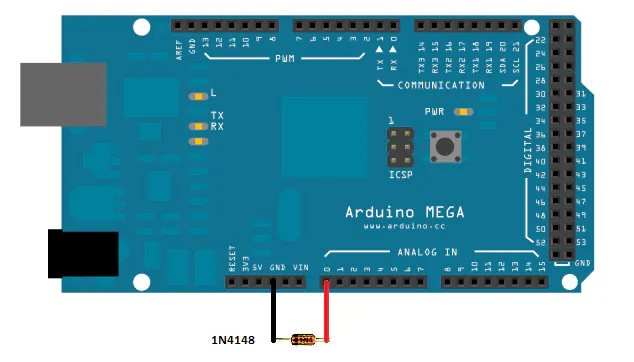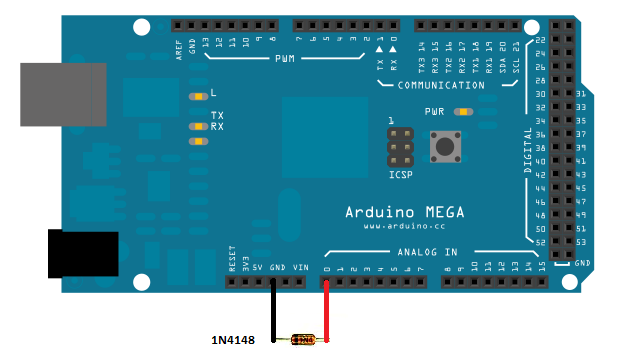How build a simple thermometer using a silicon diode

• 11,662 views
• 13 respects

Components and supplies

This project is a simple example how use the Arduino to build a thermometer using a silicon diode as temperature sensor ( i.e 1n4148 or 1n4007).

This thermometer is based on the physical caracteristic of the silicon diode that, when it is directly biased ,the voltage between the anode and catode decreas of 2.2mV /°C.

To know the temperature It is needed to measure the voltage at the terminals of the diode and compare it with the diode voltage at the room temperature.

The voltage difference is divided by the factor 0,0022 to obtain the temperature difference respect the room temperature.

if (typeof(lightBoxImages) == 'undefined') { lightBoxImages = {}; } lightBoxImages['eb3a354427'] = [{ URL: 'https://hackster.imgix.net/uploads/attachments/290201/diodotherm_nzHWw8a49o.png?auto=compress%2Cformat&w=1280&h=960&fit=max', caption: '', type: 'image' }];

Code

Diode thermometer based codeArduino
Load this code in your arduino and in the serial plot you can see the temperature read uy the diode
/*

Thermometer based on 1n4148 silicon diode used as temperature sensor.The thermometer is based on the diode characteristic that the increase of the temperature its forward voltage (VF) is lowered by 2,2mV / ° C.
Fixing the value of Vf = VF0 at ambient temperature t0, the temperature value
t is calculated with the following formula:

t= t0 - [vf(t)- vf0]* K

with K = 1 / 2,2mV

The value of Vf (t) = dtemp -vf0 is obtained by averaging values of 1024 by acquiring as many vf values

The result of t is shown on the serial monitor
*/

// set pin numbers:

const int in = A0;          // used to bias the diode  anode
const int t0 = 20.3;
const float vf0 = 573.44;
// variables will change:

int i;
float dtemp, dtemp_avg, t;

void setup() {
Serial.begin(9600);
pinMode(in, INPUT_PULLUP);            // set the  pin IN with npull up to bias the diode

}

void loop() {
dtemp_avg = 0;
for (i = 0; i < 1024; i++) {
float vf = analogRead(A0) * (4976.30 / 1023.000);
//Serial.println(vf);
dtemp = (vf - vf0) * 0.4545454;
dtemp_avg = dtemp_avg + dtemp;
}
t = t0 - dtemp_avg / 1024;
Serial.print("temperature in Celcius degree)   = " );
Serial.println(t);

delay (1000);

}

Schematics

The scheme is very simple: It is needed to connect the diode directly to the pins of the Arduino board: In particular connect the anode at the pin A0 and the catode at the GND pinApril 15, 2017

Members who respect this project

See similar projects
you might like

by microst

• 8,173 views
• 1 comment
• 4 respects

Arduino Based IR Thermometer with TFT Display and TMP006

Project tutorial by antiElectron

• 5,419 views
• 11 respects

SMS based Home Automation system using 1SHEELD

Project tutorial by Tanishq Jaiswal

• 34,863 views
• 68 respects

by Muchika

• 46,198 views
• 37 respects

DIY Art Deco Analog Thermometer

Project tutorial by Nick Koumaris

• 8,105 views